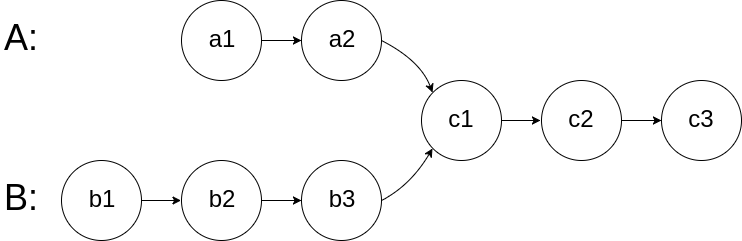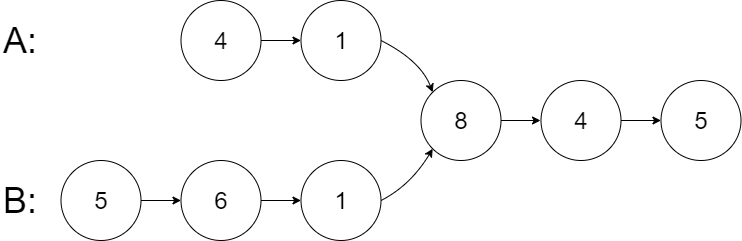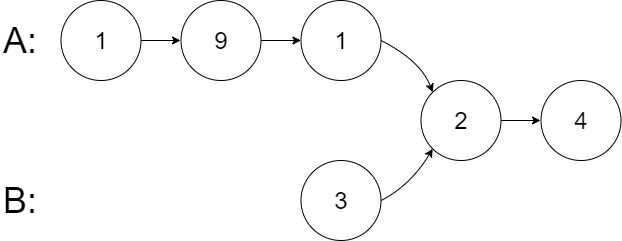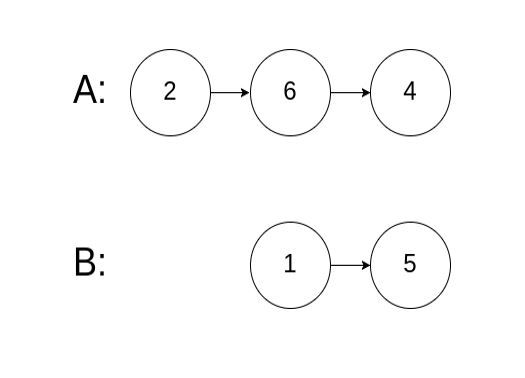3

Difficulty: MEDIUM
Avg. time to solve
25 min
Success Rate
73%

Problem Statement
Suggest Edit

#### For example:- The given Linked Lists are merging at node c1.##### Input Format:
``````The input format contains three lines consisting of three singly-linked lists.

All three lines contain the elements of the singly linked list separated by a single space and terminated by -1.

So first linked list would contain
a1, a2, ...an, c1, -1.

Similarly, the second line would contain
b1,b2, ...bm, c1, -1.

The third line would contain
c2, c3, ....ck, -1.
``````
##### Output format :
``````The only line of output contains data of the first merged node. If there is no merging output should contain -1.

You don't have to explicitly print by yourself. It has been taken care of.
``````
##### Constraints :
``````0 <= N <= 10^5
0 <= M <= 10^5
0 <= K <= 10^5.
-10^9 <= data <= 10^9 and data != -1

Where N, M, K are the size of the singly linked list respectively.

Time Limit: 1 sec
``````
##### Sample Input 1 :
``````4 1 8 -1
5 6 1 8 -1
4 5 -1
``````##### Sample Output 1 :
``````8
``````

##### Sample Input 2 :
``````1 9 1 2 -1
3 2 -1
4 -1
``````##### Sample Output 2 :
``````2
``````

##### Sample Input 3 :
``````2 6 4 -1
1 5 -1
-1
``````##### Sample Output 3 :
``````-1
``````